Area of circle and polygons to print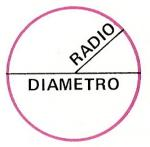Name: ______________________________________   Subject: _______________________  Date: _______

Write the missing word

1. Circumference
The segments that join the center with the circumference points are called radius.
The segment passing through the center and connects two points on the circumference is called diameter. It is equivalent to two radiuses.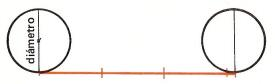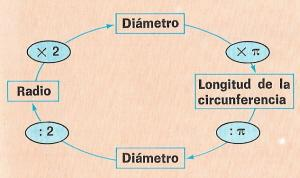If we have a coin and we paint its edge, when we move it through a paper, it will leave a mark. The length of that mark is three times the length of the diameter and a little more.
If the circumference of the coin is 44 cm and the diameter is 14.012738 cm, we find that 44:14.012738 = 3.14. Therefore the diameter fits three times in the circumference and there is an excess: 0.14. The number 3.14 is called  p (pi).
Circumference = 3.14 x length of its diameter. As the diameter is equal to two radius, we can also say that the length of the circumference = pi x 2r = 2 pi x r.  p x 2r = 2 p r.
Example: If the diameter of a circumference is 16 cm, its length will be: 3.14 x 16 = 50.24 cm.

Do these problems:

 Find the length in cm of a bicycle wheel that is 50 cm radius. The length of a ring is 14 dm. How many dm is the radius? A tree is 1.5 m in perimeter. What is its diameter? Find the length in meters of a bullring which is 116 m in diameter.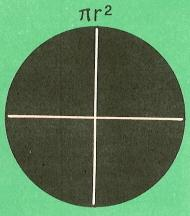2. - Area of a Circle
The formula for calculating the area of a circle = pi x r2.
Example: If a circle is 8 m radius, its area will be pi x 82 = 3.14 x 8 x 8 = 200.96 m2.
Do these exercises on a paper and answer in cm2:

 A circle is 9 cm radius. What is its area in cm2 ? A circle is 60 cm in diameter. How many cm2 is it? Calculate the area in cm2 of a circle that is 25 cm radius. Calculate the area in m2 of a circle of 7 m in diameter.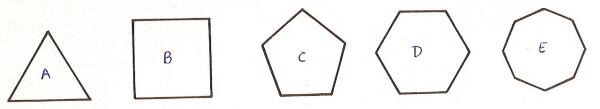3. Regular Polygons
The polygons are regular if all its sides are equal.
How do you call these regular polygons?

 What do you call the A? What do you call the C? What do you call the B? What do you call the E? What do you call the D?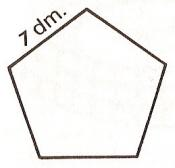4. Perimeter of regular polygon
The perimeter is the sum of all its sides. In the picture, the side of the pentagon is 7 dm. The perimeter is 7 +7 + 7 + 7 + 7 = 35 dm; perimeter = 7 x 5 = 35 dm. Perimeter = side x number of sides.
 The side of a regular hexagon is 5 cm. How many cm is its perimeter? An equilateral triangle has a perimeter of 15 meters. How long is one of its sides? An octagon side is 3 cm. How many cm is its perimeter? What is the perimeter of a square that is 22 m side?5. Area of regular polygons
This regular hexagon is divided into 6 triangles. The triangle ABO has as height the segment A. This segment is called apothem of the polygon.
The area of each triangle is the product of the side by the apothem divided by 2, s x/2.
As we have 6 triangles, the area of the hexagon is 6 x s x a / 2.
The area of the regular polygon is equal to half the product of the apothem perimeter.

| Educational applications | Mathematics |
In Spanish  | Interactive

®Arturo Ramo García.-Record of intellectual property of Teruel (Spain) No 141, of 29-IX-1999
Plaza Playa de Aro, 3, 1ş DO 44002-TERUEL